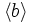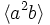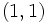# Subgroup structure of generalized quaternion group:Q16

## Contents

View subgroup structure of particular groups | View other specific information about generalized quaternion group:Q16

The generalized quaternion group:Q16, denoted$Q_{16}$is a group of order$16$, is a generalized quaternion group. It can be described by the following presentation:$G := \langle a,b,c \mid a^4 = b^2 = c^2 = abc \rangle$.

Note that$c = ab = ba^{-1}$ from these relations, and$bab^{-1} = a^{-1}$. This in turn forces that$b^2 = b(b^2)b^{-1} = ba^4b^{-1} = a^{-4} = b^{-2}$, forcing$b^2 = a^4 = c^2 = abc$ to have order two. We shall denote this element of order two, which is clearly central, as$z$.

Here is a list of subgroups:

1. The trivial subgroup. Isomorphic to trivial group. (1)
2. The center, which is a subgroup of order two, generated by$z = a^4 = b^2 = c^2$. Isomorphic to cyclic group:Z2. (1)
3. The cyclic subgroup of order four generated by$a^2$. Isomorphic to cyclic group:Z4. (1)
4. The four cyclic subgroups of order four, namely:$\langle b \rangle$,$\langle ab \rangle$,$\langle a^2b \rangle$ and$\langle a^3b\rangle$. These come in two conjugacy classes of 2-subnormal subgroups, one conjugacy class comprising$\langle ab \rangle$ and$\langle a^3b \rangle$ and the other comprising$\langle b \rangle$ and$\langle a^2b \rangle$. Isomorphic to cyclic group:Z4. (4)
5. The cyclic subgroup of order eight, generated by$a$. This is characteristic; in fact, it equals the centralizer of commutator subgroup. Isomorphic to cyclic group:Z8. (1)
6. Two quaternion groups of order eight, namely$\langle a^2,b \rangle$ and$\langle a^2, ab \rangle$. Isomorphic to quaternion group. (2)
7. The whole group. (1)

### Table classifying isomorphism types of subgroups

Group name GAP ID Occurrences as subgroup Conjugacy classes of occurrence as subgroup Occurrences as normal subgroup Occurrences as characteristic subgroup
Trivial group$(1,1)$ 1 1 1 1
Cyclic group:Z2$(2,1)$ 1 1 1 1
Cyclic group:Z4$(4,1)$ 5 3 1 1
Cyclic group:Z8$(8,1)$ 1 1 1 1
Quaternion group$(8,4)$ 2 2 2 0
Generalized quaternion group:Q16$(16,9)$ 1 1 1 1
Total -- 11 9 7 5

### Table listing number of subgroups by order

Group order Occurrences as subgroup Conjugacy classes of occurrence as subgroup Occurrences as normal subgroup Occurrences as characteristic subgroup$2$ 1 1 1 1$2$ 1 1 1 1$4$ 5 3 1 1$8$ 3 3 3 1$16$ 1 1 1 1
Total 11 9 7 5